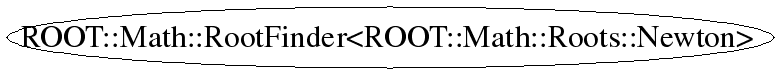# class ROOT::Math::RootFinder<ROOT::Math::Roots::Newton>

```
Class to find the Root of one dimensional functions.
The class is templated on the type of Root solver algorithms.
The possible types of Root-finding algorithms are:
<ul>
<li>Root Bracketing Algorithms which they do not require function derivatives
<ol>
<li>Roots::Bisection
<li>Roots::FalsePos
<li>Roots::Brent
</ol>
<li>Root Finding Algorithms using Derivatives
<ol>
<li>Roots::Newton
<li>Roots::Secant
<li>Roots::Steffenson
</ol>
</ul>

This class does not cupport copying

@ingroup RootFinders

```

## Function Members (Methods)

public:
 virtual ~RootFinder() int Iterate() int Iterations() const const char* Name() const double Root() const ROOT::Math::RootFinder RootFinder() int SetFunction(const ROOT::Math::IGradFunction& f, double Root) int SetFunction(const ROOT::Math::IGenFunction& f, double xlow, double xup) int Solve(int maxIter = 100, double absTol = 1E-3, double relTol = 1E-6) static int TestDelta(double r1, double r0, double epsAbs, double epsRel) static int TestInterval(double xlow, double xup, double epsAbs, double epsRel) static int TestResidual(double f, double epsAbs)
private:
 ROOT::Math::RootFinder& operator=(const ROOT::Math::RootFinder& rhs) ROOT::Math::RootFinder RootFinder(const ROOT::Math::RootFinder&)

## Data Members

private:
 ROOT::Math::Roots::Newton fSolver type of algorithm to be used

## Class Charts## Function documentation

int SetFunction(const ROOT::Math::IGenFunction& f, double xlow, double xup)
```Provide to the solver the function and the initial search interval [xlow, xup]
for algorithms not using derivatives (bracketing algorithms)
The templated function f must be of a type implementing the \a operator() method,
<em>  double  operator() (  double  x ) </em>
Returns non zero if interval is not valid (i.e. does not contains a root)

```
return fSolver. SetFunction(const ROOT::Math::IGenFunction& f, double xlow, double xup)
int Solve(int maxIter = 100, double absTol = 1E-3, double relTol = 1E-6)
```Compute the roots iterating until the estimate of the Root is within the required tolerance returning
the iteration Status

```
int Iterations()
```Return the number of iteration performed to find the Root.

```
int Iterate()
```Perform a single iteration and return the Status

```
double Root()
```Return the current and latest estimate of the Root

```
const char * Name()
```Return the current and latest estimate of the lower value of the Root-finding interval (for bracketing algorithms)

double XLower() const {
return fSolver.XLower();
}

Return the current and latest estimate of the upper value of the Root-finding interval (for bracketing algorithms)

double XUpper() const {
return  fSolver.XUpper();
}

Get Name of the Root-finding solver algorithm

```
int TestInterval(double xlow, double xup, double epsAbs, double epsRel)
```Test convertgence Status of current iteration using interval values (for bracketing algorithms)

```
int TestDelta(double r1, double r0, double epsAbs, double epsRel)
```Test convergence Status of current iteration using last Root estimates (for algorithms using function derivatives)

```
int TestResidual(double f, double epsAbs)
```Test function residual

```

Last update: root/mathmore:\$Id: RootFinder.h 21503 2007-12-19 17:34:54Z moneta \$
Copyright (c) 2004 ROOT Foundation, CERN/PH-SFT *

This page has been automatically generated. If you have any comments or suggestions about the page layout send a mail to ROOT support, or contact the developers with any questions or problems regarding ROOT.